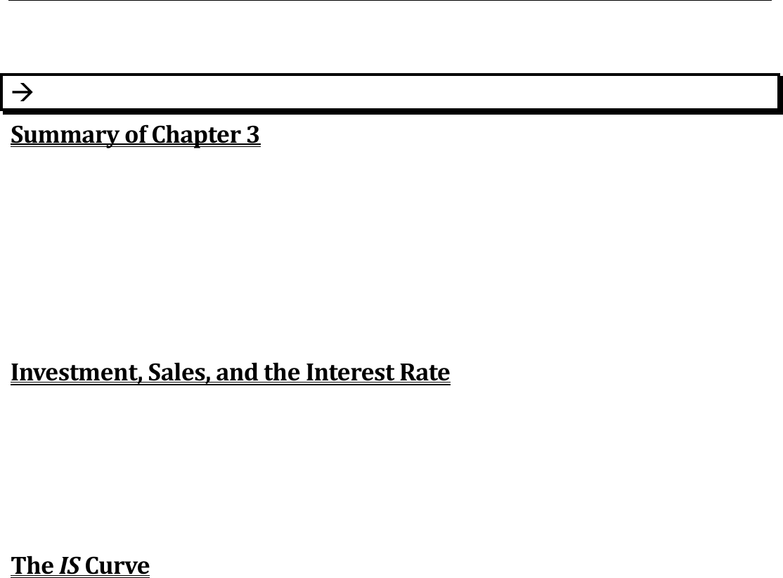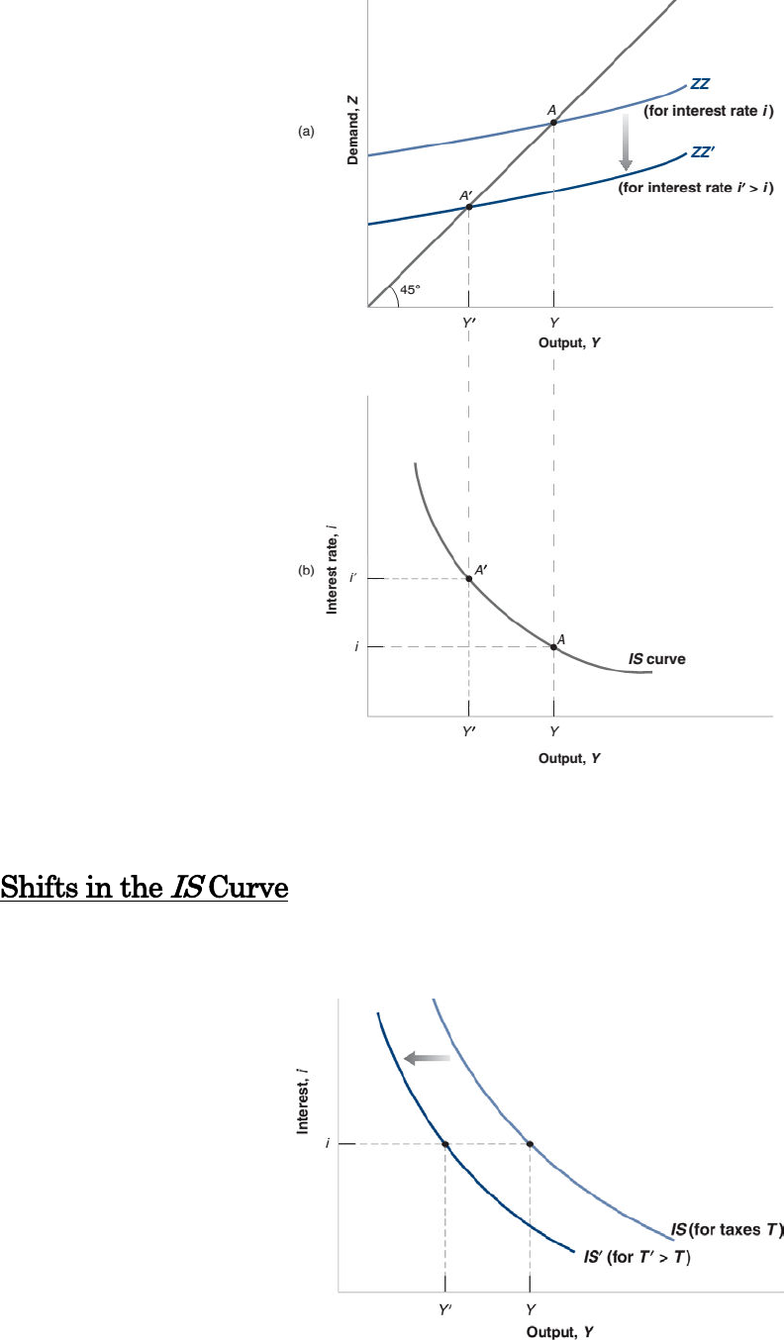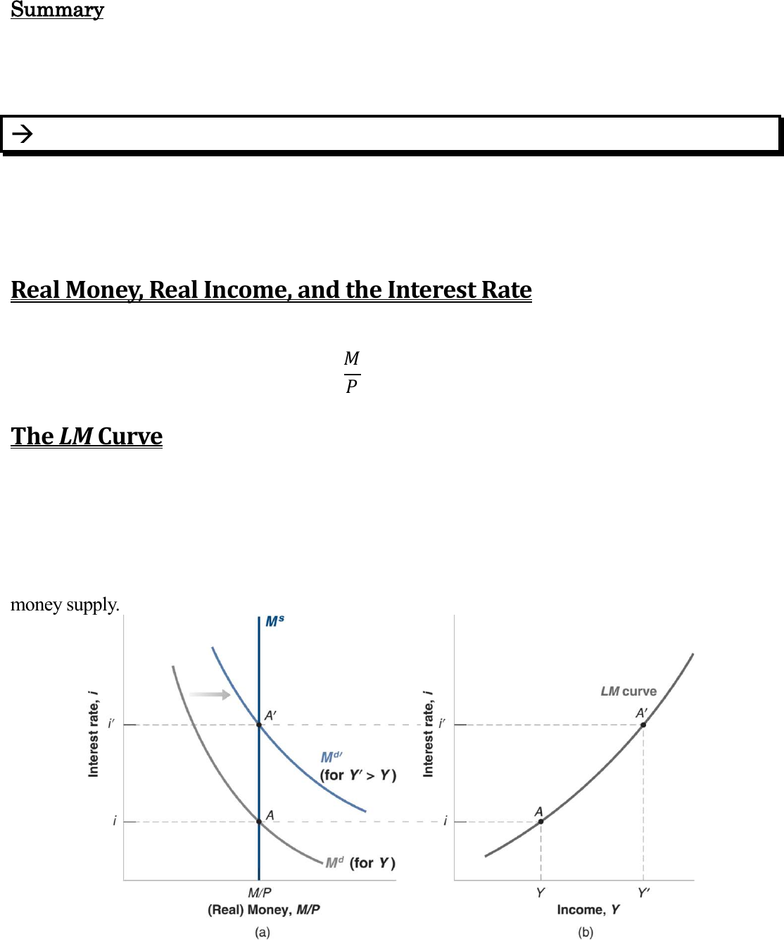Textbook Notes (280,000)
CA (160,000)
UTM (8,000)
ECO (400)
Chapter 5

# ECO202Y5 Chapter Notes - Chapter 5: Exogeny, Open Market Operation, Disposable And Discretionary Income

Department
Economics
Course Code
ECO202Y5
Professor
Michael Ho
Chapter
5

This preview shows pages 1-2. to view the full 8 pages of the document.Prof. Michael Ho ECO202 Page 1 of 8
Chapter 5 Goods & Financial Markets: The IS-LM Model
Chapter 5 Goods & Financial
Markets: The IS-LM Model
Goods Market and IS Relation; Financial Markets and LM Relation; IS-LM Model; Using
Policy; LM Relation When the Central Bank Directly Targets the Interest Rate
5-1: THE GOODS MARKET AND THE IS RELATION
Summary of Chapter 3
o Equilibrium in the goods market: Y = Z
o The equality of demand and production is called the IS relation because it has been historically
reinterpreted as the equality of I (investment) and S (saving).
o Z = C + I + G
o Consumption is a function of disposable income (income minus taxes). We took I, G, and T as
given. Equilibrium condition was given by:
=( − )++
Previously, we had 2 simplifications: (1) interest rates do not affect demand, and (2) exports &
imports were 0. In this chapter, we will remove the first simplification.
Investment, Sales, and the Interest Rate
Investment depends primarily on 2 factors:
o Level of Sales. A firm facing an increase in sales needs to increase production.
o Interest Rate. When a firm needs to buy a new machine (invest), the firm must borrow either by
taking a loan or issuing bonds. The higher the interest rate, the less likely the firm is to borrow.
To capture these two effects, we write the investment relation as follows:
=(,)
(+, )
The IS Curve
Considering the investment relation, the equilibrium condition in the goods market becomes:
=( − )+(,)+
Equilibrium is reached at the point where demand equals production, at point Athe intersection of
ZZ and the 45° line. The equilibrium level of output is given by Y. Suppose the interest rate increases
exogenously. At any level of output, investment decreases. ZZ shifts down. Equilibrium is now Y '.
The initial decrease in investment leads to a larger decrease in output through the multiplier effect.
Using ZZ, we can find the equilibrium value of output associated with any value of the interest rate.
Next page.

Only pages 1-2 are available for preview. Some parts have been intentionally blurred.Prof. Michael Ho ECO202 Page 2 of 8
Chapter 5 Goods & Financial Markets: The IS-LM Model
Generally, equilibrium in the goods market implies that the higher the interest rate, the lower is the
equilibrium level of output. This relation between the interest rate and output is represented by the
downward-sloping curve called the IS curve.
Shifts in the
IS
Curve
Consider an increase in taxes. At a given interest rate, consumption decreases, leading to a decrease
in the demand for goods and (through the multiplier) to a decrease in equilibrium output. The IS
curve shifts to the left: at any interest rate, the equilibrium level of output is lower than before.
Generally, any factor that (for a given interest rate) decreases the equilibrium level of output, leads
the IS curve to shift to the left. Ex: decrease in G, decrease in consumer confidence.
The same is true of the opposite; increases in equilibrium level of output shifts the IS curve right.

Unlock to view full version

Only pages 1-2 are available for preview. Some parts have been intentionally blurred.Prof. Michael Ho ECO202 Page 3 of 8
Chapter 5 Goods & Financial Markets: The IS-LM Model
Summary
o Equilibrium in the goods market implies that output is a decreasing function of the interest rate.
o This relation is represented by the downward-sloping IS curve.
o Changes in factors that decrease or increase the demand for goods given the interest rate shift the
IS curve to the left or to the right.
5-2: FINANCIAL MARKETS AND THE LM RELATION
The interest rate is determined by the equality of the supply and demand of money.
= \$ ()
M is the nominal money supply. The right side is the demand for money, which is a function of
nominal income (\$Y) and the nominal interest rate i. An increase in the nominal income increases the
demand for money, and an increase in the interest rate decreases the demand for money.
Real Money, Real Income, and the Interest Rate
Recall that nominal income divided by the price level equals real income, Y. Dividing both sides of
the equation by the price level P gives:
= ()
This is the LM relation. The real money supply equal to the real money demand.
The LM Curve
Let the interest rate be measured on the vertical axis and (real) money be measured on the horizontal
axis. Money supply is given by the vertical line at M/P and is denoted M s. Money demand is a
decreasing function of the interest rate (for a given level of income Y) and is, therefore, a downward-
sloping curve denoted M d.
Consider an increase in income, which increases demand for money at any given interest rate. Using
(a), we can find out the value of the interest rate associated with any value of income for a given
money supply.
Generally, equilibrium in financial markets implies that the higher the level of output, the higher the
demand for money and therefore the higher is the equilibrium interest rate. This relation between
output and the interest rate is represented by the upward-sloping curve called the LM curve.